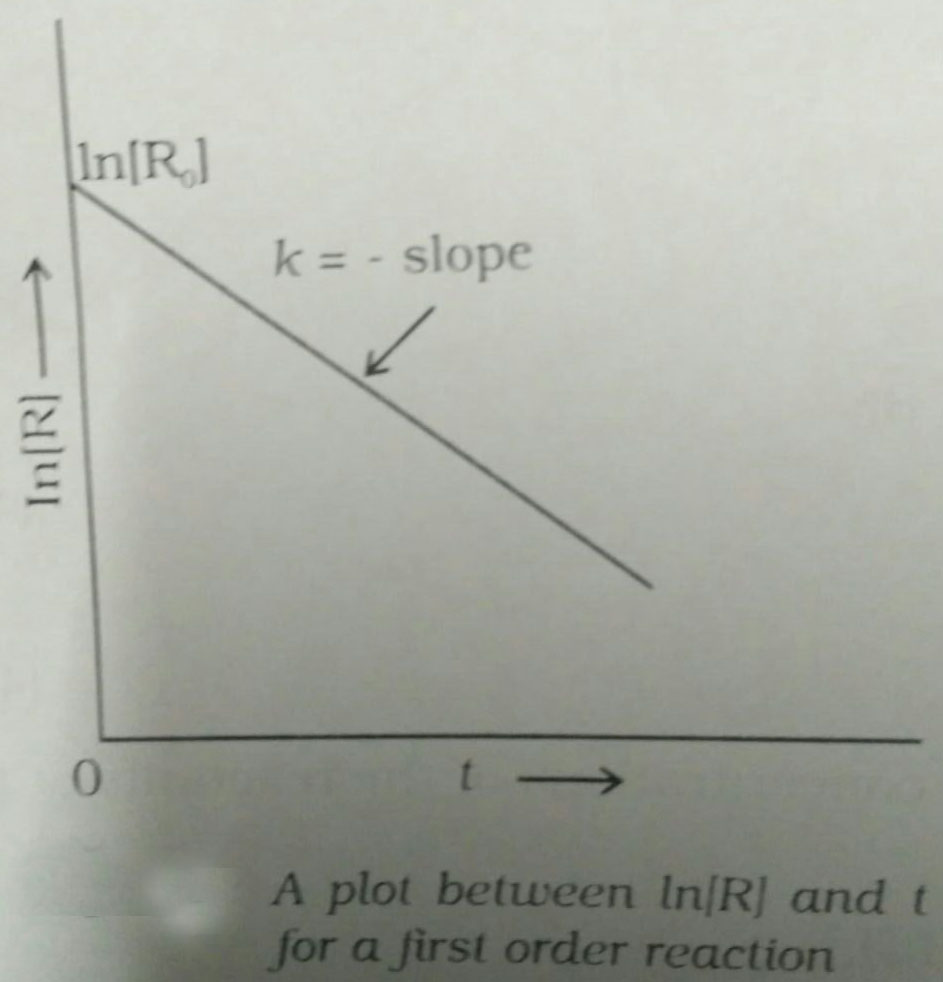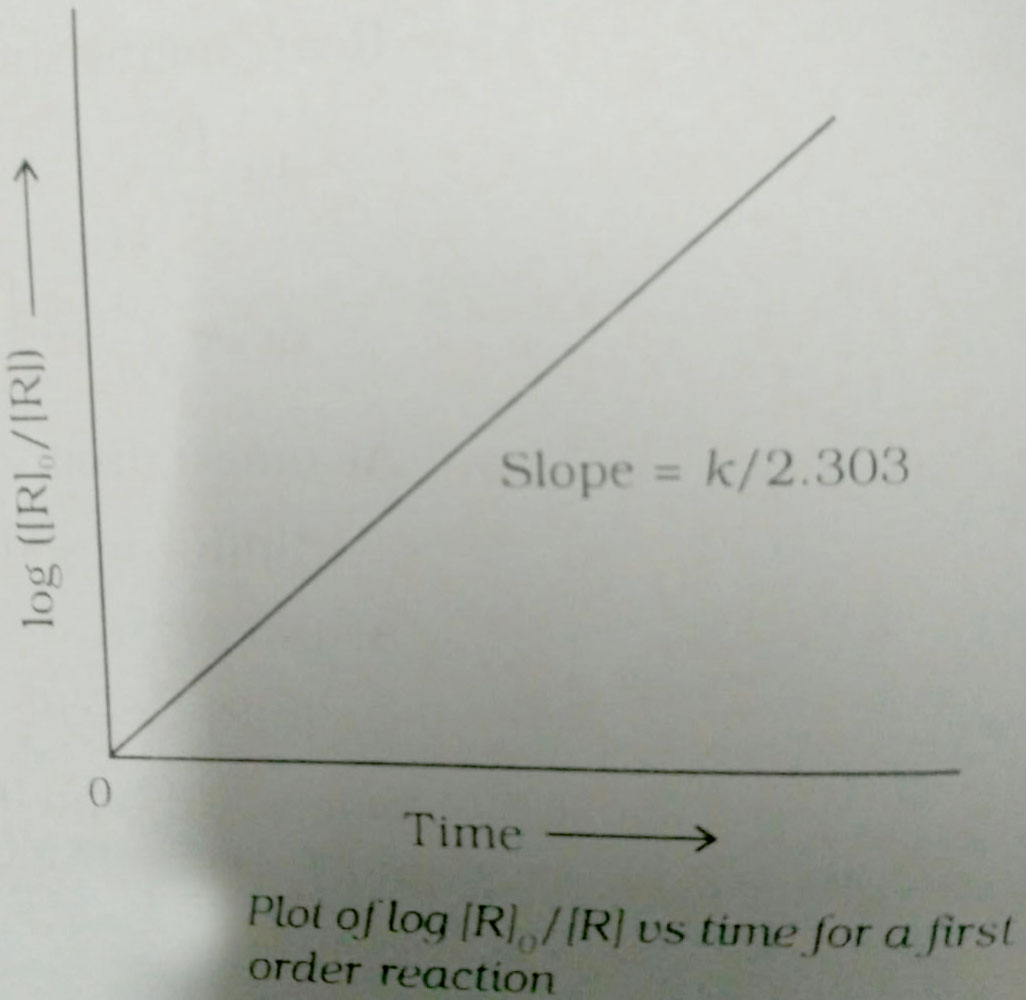# A first order reaction is 50% completed in. How much time would it take for 100% completion? Option 1)Option 2)Option 3)Option 4) Infinite

As we learned in concept

First Order Reaction -

The rate of the reaction is proportional to the first power of the concentration of the reaction- wherein

Formula:

RP

a                 0

a-x             x[differentiate rate law]Unit ofFirst Order Reaction -

The rate of the reaction is proportional to the first power of the concentration of the reaction- wherein

Formula:

RP

a                 0

a-x             x[differentiate rate law]Unit ofIn a first order reaction,Rate =, k= constantGiven that  at x= a/2,but for 100% completion, x=a and that would render equation 1 as undefined.

So,first order reactions take infinite time to complete.

Option 1)Incorrect option

Option 2)Incorrect option

Option 3)Incorrect option

Option 4)

Infinite

Correct option

Boost your Preparation for JEE Main 2021 with Personlized Coaching

Exams
Articles
Questions